#### Fay’s BRR Method

Fay’s method is a modification of the BRR method, and it requires a stratified sample design with two primary sampling units (PSUs) per stratum. The total number of replicates R is the smallest multiple of 4 that is greater than the total number of strata H. However, if you prefer a larger number of replicates, you can specify the REPS= method-option.

For each replicate, Fay’s method uses a Fay coefficient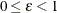to impose a perturbation of the original weights in the full sample that is gentler than using only half-samples, as in the traditional BRR method. The Fay coefficientcan be set by specifying the FAY =method-option. By default,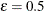if the FAY method-option is specified without providing a value for(Judkins, 1990; Rao and Shao, 1999). When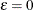, Fay’s method becomes the traditional BRR method. For more details, see Dippo, Fay, and Morganstein (1984); Fay (1984, 1989); Judkins (1990).

Let H be the number of strata. Replicates are constructed by using the first H columns of the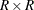Hadamard matrix, where R is the number of replicates,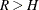. The rth (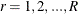) replicate is created from the full sample according to the rth row of the Hadamard matrix as follows:

• If the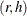element of the Hadamard matrix is 1, then the full sample weight of the first PSU in stratum h is multiplied byand the full sample weight of the second PSU is multiplied byto obtain the rth replicate weights.

• If theelement of the Hadamard matrix is –1, then the full sample weight of the first PSU in stratum h is multiplied byand the full sample weight of the second PSU is multiplied byto obtain the rth replicate weights.

You can use the VARMETHOD=BRR(OUTWEIGHTS=) method-option to save the replicate weights into a SAS data set.

By default, an appropriate Hadamard matrix is generated automatically to create the replicates. You can request that the Hadamard matrix be displayed by specifying the VARMETHOD=BRR(PRINTH) method-option. If you provide a Hadamard matrix by specifying the VARMETHOD=BRR(HADAMARD=) method-option, then the replicates are generated according to the provided Hadamard matrix.

Let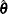be the estimated regression coefficients from the full sample for. Let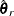be the estimated regression coefficient obtained from the rth replicate by using replicate weights. PROC SURVEYLOGISTIC estimates the covariance matrix ofby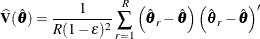with H degrees of freedom, where H is the number of strata.